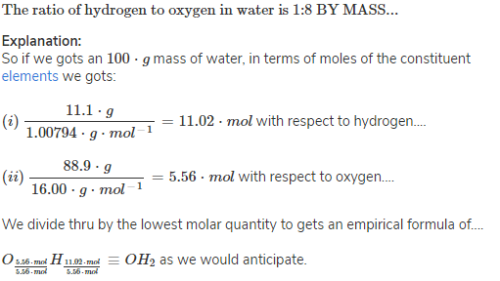# Atoms & Molecules - Practice Test, Class 9 Science

## 20 Questions MCQ Test | Atoms & Molecules - Practice Test, Class 9 Science

Description
This mock test of Atoms & Molecules - Practice Test, Class 9 Science for Class 9 helps you for every Class 9 entrance exam. This contains 20 Multiple Choice Questions for Class 9 Atoms & Molecules - Practice Test, Class 9 Science (mcq) to study with solutions a complete question bank. The solved questions answers in this Atoms & Molecules - Practice Test, Class 9 Science quiz give you a good mix of easy questions and tough questions. Class 9 students definitely take this Atoms & Molecules - Practice Test, Class 9 Science exercise for a better result in the exam. You can find other Atoms & Molecules - Practice Test, Class 9 Science extra questions, long questions & short questions for Class 9 on EduRev as well by searching above.
QUESTION: 1

### Which of the following does not represent molar mass of a substance?

Solution:

Mas of oxygen O1 is 16 and the molar mass of O2 will be 16×2=32gm

QUESTION: 2

Solution:
QUESTION: 3

### Which of the following does not change when a chemical reaction takes place?

Solution:
QUESTION: 4
The quantity of matter present in an object is called its _________.
Solution:
QUESTION: 5
A sample contains 22 g of carbon dioxide. This is equal to?
Solution:
QUESTION: 6
An example of a triatomic molecule is ___________.
Solution:
QUESTION: 7

Formula mass is not used in case of -

Solution:
QUESTION: 8
All samples of carbon dioxide contain carbon and oxygen in the mass ratio of 3:8. This is in agreement with the Law of ___________.
Solution:
QUESTION: 9
In 16O8, the number 16 stands for?
Solution:
QUESTION: 10
The number of moles in 5 grams of calcium is _________.
Solution:
QUESTION: 11

The smallest particle of a substance that is capable of independent existence is _________.

Solution:
QUESTION: 12
Atomic mass of calcium is 40. The mass of 2.5 gram atoms of calcium is _______.
Solution:
QUESTION: 13
Molecules without a charged valency are contained by only?
Solution:
QUESTION: 14
Kalium is the Latin name of ________.
Solution:
QUESTION: 15
The number of atoms in a molecule of the elementary substance is called ________.
Solution:
QUESTION: 16
An atom is 15 times heavier than 1/12th of the mass of Carbon atom (C-12 isotopes). The mass in a.m.u is?
Solution: The scientists had taken a standard unit to calculate the mass of atom. So they have taken the carbon 12 atoms as a unit to find the atomic mass. But it was very difficult to write always 1/12TH CARBON 12 ATOM.So instead they write it as amu(atomic mass unit) which was taken as 12 units also any mass of any
atom is always x times greater than the amu. So in this case the answer is 15amu.
QUESTION: 17
In water, hydrogen and oxygen are present in the ratio of ________.
Solution:QUESTION: 18
Indivisibility of an atom was proposed by ___________.
Solution:
QUESTION: 19
The molecular mass of ammonia is _________.
Solution:
QUESTION: 20
The atomic mass of sodium is 23. The number of moles in 46g of sodium is ________.
Solution: